Sparse Matrix Techniques

A key component of the HPMIXED procedure is the use of sparse matrix techniques for computing and optimizing the likelihood expression given in the section Model Assumptions. There are two aspects to sparse matrix techniques, namely, sparse matrix storage and sparse matrix computations. Typically, computer programs represent an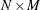matrix in a dense form as an array of size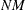, making row-wise and column-wise arithmetic operations particularly efficient to compute. However, if many of thesenumbers are zeros, then correspondingly many of these operations are unnecessary or trivial. Sparse matrix techniques exploit this fact by representing a matrix not as a complete array, but as a set of nonzero elements and their location (row and column) within the matrix. Sparse matrix techniques are more efficient if there are enough zero-element operations in the dense form to make the extra time required to find and operate on matrix elements in the sparse form worthwhile.

The following discussion illustrates sparse techniques. Let the symmetric matrix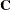be the matrix of mixed model equations of size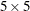.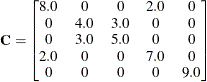There are 15 elements in the upper triangle of, though eight of them are zeros. The row and column indices and the values of seven nonzero elements are listed as follows:

 i 1 1 2 2 3 4 5 j 1 4 2 3 3 4 5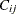8 2 4 3 5 7 9

The most elegant scheme to store these seven elements is to store them in a hash table with row and column indices as a hash key. However, this scheme is not efficient as the number of non-zero elements gets very large. The classical and widely used scheme, and the one the HPMIXED procedure employs, is the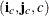format, in which the nonzero elements are stored contiguously row by row in the vector c. To identify the individual nonzero elements in each row, you need to know the column index of an element. These column indices are stored in the vector
; that is, if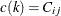, then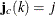. To identify the individual rows, you need to know where each row starts and ends. These row starting positions are stored in the vector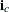. For instance, ifis the first nonzero element in the row i and, then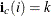. The row i ending position is one less than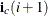. Thus, the number of nonzero elements in the row i is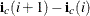, these elements in the row i are stored consecutively starting from the position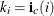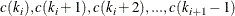and the corresponding columns indices are stored consecutively in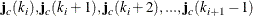For example, the seven nonzero elements in matrixare stored informat as1 3 5 6 7 81 4 2 3 3 4 5 c 8 2 4 3 5 7 9.0

Note that since matrices are stored row by row in theformat, row-wise operations can be performed efficiently but it is inefficient to retrieve elements column-wise. Thus, this representation will be inefficient for matrix computations requiring column-wise operations. Fortunately, the likelihood calculations for mixed models can usually avoid column-wise operations.

In mixed models, sparse matrices typically arise from a large number of levels for fixed effects and/or random effects. If a linear model contains one or more large CLASS effects, then the mixed model equations are usually very sparse. Storing zeros in mixed model equations not only requires significantly more memory but also results in longer execution time and larger rounding error. As an illustration, the example in the Getting Started: HPMIXED Procedure has 3506 mixed model equations. Storing just the upper triangle of these equations in a dense form requires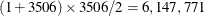elements. However, there are only 60,944 nonzero elements—less than 1% of what dense storage requires.

Note that as the density of the mixed model equations increases, the advantage of sparse matrix techniques decreases. For instance, a classical regression model typically has a dense coefficient matrix, though the dimension of the matrix is relatively small.

The HPMIXED procedure employs sparse matrix techniques to store the nonzero elements in the mixed model equations and to compute a sparse Cholesky decomposition of these equations. A reordering of the mixed model equations is required in order to keep the minimum memory consumption during the factorization. This reordering process results in a different g-inverse from what is produced by most other SAS/STAT procedures, for which the g-inverse is defined by sequential sweeping in the order defined by the model. If mixed model equations are singular, this different g-inverse produces a different solution of mixed model equations. However, estimable functions and tests based on them are invariant to the choice of g-inverse, and are thus the same for the HPMIXED procedure as for other procedures.